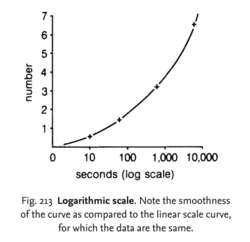# logarithmic scale

(redirected from Logarithmic measure)
Also found in: Dictionary, Thesaurus, Financial, Encyclopedia.Fig. 213 Logarithmic scale . Note the smoothness of the curve as compared to the linear scale curve, for which the data are the same.

## logarithmic scale

a scale in which the values of a variable are expressed as logarithms. Such transformations of data are often employed to simplify the drawing of lines on a graph, where the variable values are spread over a wide range. See Fig. 213 . A good example of the use of log scales is seen in Fig. 182 where the LOG PHASE of a microbial GROWTH CURVE is illustrated. Compare LINEAR SCALE.
Collins Dictionary of Biology, 3rd ed. © W. G. Hale, V. A. Saunders, J. P. Margham 2005
References in periodicals archive ?
Suppose that there exists some subset E [subset] [R.sup.+] with logarithmic measure log mesE < +[infinity], such that
From (3.70) and Lemma 2.5 we know that there exists some subset E [subset] (1, [infinity]) with finite logarithmic measure, i.e., [[integral].sub.E] dt/t < [infinity], such that
Then there exist a set [E.sub.1] [subset] (1, +[infinity]) having finite logarithmic measure and a constant B > 0 that depends only on [sigma] and (i, j) (i, j positive integers with i > j) such that for all z satisfying [absolute value of z] = r [not member of] [0,1] [union] [E.SUB.1], we have
Then there exist a sequence [{[r.sub.m]}.sub.m[member of]N], [r.sub.m] [right arrow] +[infinity] and a set [E.sub.2] of finite logarithmic measure such that the estimation
Then for any given [epsilon] > 0, there exists a set [E.sub.3] [subset] (1, +[infinity]) that has finite logarithmic measure, such that
Then for any given [epsilon] > 0, there exists a set E [subset] (1, [infinity]) of [absolute value of z] = r of finite logarithmic measure, so that
Suppose that f (z) is a transcendental entire function with finite order [sigma](f), and a set E [subset] (1, [infinity]) has a finite logarithmic measure and G = {[[phi].sub.1], ..., [[phi].sub.n]} [subset] [0, 2[pi]).
By Lemma 2.4 and its Remark 2.1, for any given 0 < [epsilon] < 1/2, there exists a set [E.sub.1] [member of] (1, [infinity]) of finite logarithmic measure, such that for all z satisfying [absolute value of z] = r [not member of] [0, 1] [union] [E.sub.1] and r sufficiently large, we have

Site: Follow: Share:
Open / Close